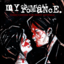MathematicsOpenStudy (anonymous):

Determine the zeros of f(x) = x3 – 12x2 + 28x – 9 help please:)OpenStudy (amistre64):

1 -12 28 -9 0 <-- add these ------------- x ) 1 <- multiply these your "x" will be whatever you wanna test for a zeroOpenStudy (amistre64):

1,3,9 loos to be our optionsOpenStudy (amistre64):

1 -12 28 -9 0 3 -27 3 ------------- 3 ) 1 -9 1 -6: -6 is NOT a zero so "3" is outOpenStudy (amistre64):

1 -12 28 -9 0 -3 45 ------------- -3 ) 1 -15 ...... this aint gonna zero out eitherOpenStudy (amistre64):

1 -12 28 -9 0 9 -27 9 ------------- 9 ) 1 -3 1 0 : x=9 works as a zeroOpenStudy (amistre64):

x^2 -3x +1 is a quadratic form now that can be done with the quad formula if need beOpenStudy (anonymous):

thank you!OpenStudy (anonymous):

If guess that one of the roots is rational, the possible roots from the rational root theorem are ±1 , ±3 , ±9. If I try x = 9, I find that f(9) = 0. x^3 - 12x^2 + 28x - 9 = (x-9)(x^2 - 3x + 1) Using the quadratic formula, the other 2 solutions are (3 ± sqrt(9-4))/2 = (3 ± sqrt(5))/2 Solutions to f(x) = 0 : 9 , (3 + sqrt(5))/2 , (3 - sqrt(5))/2OpenStudy (amistre64):

youre welcome :)OpenStudy (anonymous):

understand?OpenStudy (anonymous):

yes thank you:)

Latest Questionshayhay6: Give an example of an equation of a line with an undefined slope. What type of li
22 minutes ago 1 Reply 0 Medalshayhay6: What is the equation of a line that passes through the point (5, -3) and has a sl
33 minutes ago 1 Reply 0 Medalspiperkoebel: Does anyone know the coordinates of two points on the line y = u22122?
35 minutes ago 1 Reply 0 Medalslolokrat: anyone good with microeconomics that can help me find out where i went wrong on m
11 hours ago 0 Replies 0 MedalsAsleepAndUnafraid21: URGENT PLZ HELP NOW Part C: Find the distance from B to E and from P to E. Show your work.
13 hours ago 49 Replies 5 MedalsKachirikutoka: How many solutions does the following equation have? |3x + 12| = 18 a No solution
17 hours ago 1 Reply 1 MedalAsleepAndUnafraid21: In the figure below, segment DE is parallel to segment BC and segment EF is parallel to AB: The figure shows triangle ABC with segments DE and DF.
14 hours ago 11 Replies 0 MedalsAsleepAndUnafraid21: In the figure below, segment DE is parallel to segment BC and segment EF is parallel to AB: The figure shows triangle ABC with segments DE and DF.
18 hours ago 1 Reply 0 Medalslakeawilliams: What is the solution to StartFraction 5 over 6 EndFraction x minus one-third grea
20 hours ago 1 Reply 0 Medalskendall22: Identify the sentence that uses modifiers in the correct form. A. The food looked great but tasted awfully.
19 hours ago 1 Reply 0 Medals